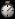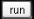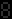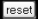Go to content
2 marksThis display is based on a typical examination question. You can change some of the details in the question and then see the answer, step by step.You can get a better display of the maths by downloading special TeX fonts from jsMath. In the meantime, we will do the best we can with the fonts you have, but it may not be pretty and some equations may not be rendered correctly.

Glossary

union

The union of two sets A and B is the set containing all the elements of A and B.

Full Glossary List

This question appears in the following syllabi:

SyllabusModuleSectionTopicExam Year
AQA GCSE (9-1) Foundation (UK)P: ProbabilityP1: Frequencies in Tables and TreesBasic Probability Problems-
CBSE IX (India)ProbabilityExperimentsRepeated experiments-
CBSE X (India)Statistics and ProbabilityProbabilityDefinition-
CIE IGCSE (9-1) Maths (0626 UK)8 ProbabilityC8.1 Calculating a ProbabilityBasic Probability Problems-
Edexcel GCSE (9-1) Foundation (UK)P: ProbabilityP1: Frequencies in Tables and TreesBasic Probability Problems-
GCSE Foundation (UK)ProbabilityProbabilityBasic problems-
OCR GCSE (9-1) Foundation (UK)11: Probability11.01d: Equally Likely Outcomes and ProbabilityBasic Probability Problems-
Universal (all site questions)PProbabilityBasic problems-